• ## 协方差公式推导

万次阅读 2017-05-24 14:57:47
协方差公式推导cov(X,Y)=∑ni=1(Xi−X¯)(Yi−Y¯)n=E[(X−E[X])(Y−E[Y])] cov(X,Y)=\frac{\sum_{i=1}^{n}(X_i-\bar{X})(Y_i-\bar{Y})}{n}=E[(X-E[X])(Y-E[Y])] =E[XY−E[X]Y−XE[Y]+E[X]E[Y]] =E[XY-E[X]Y-XE[Y]+...
协方差公式推导

cov(X,Y)=∑ni=1(Xi−X¯)(Yi−Y¯)n=E[(X−E[X])(Y−E[Y])]<!--//--><![CDATA[//><!--
cov(X,Y)=\frac{\sum_{i=1}^{n}(X_i-\bar{X})(Y_i-\bar{Y})}{n}=E[(X-E[X])(Y-E[Y])]
//--><!]]>=E[XY−E[X]Y−XE[Y]+E[X]E[Y]]<!--//--><![CDATA[//><!--
=E[XY-E[X]Y-XE[Y]+E[X]E[Y]]
//--><!]]>
因为均值计算是线性的，即（a和b均为常数）： E[aX+bY]=aE[X]+bE[Y]<!--//--><![CDATA[//><!--
E[aX+bY]=aE[X]+bE[Y]
//--><!]]>
则我们有： E[XY−E[X]Y−XE[Y]+E[X]E[Y]]<!--//--><![CDATA[//><!--
E[XY-E[X]Y-XE[Y]+E[X]E[Y]]
//--><!]]>=E[XY]−E[X]E[Y]−E[X]E[Y]+E[X]E[Y]<!--//--><![CDATA[//><!--
=E[XY]-E[X]E[Y]-E[X]E[Y]+E[X]E[Y]
//--><!]]>=E[XY]−E[X]E[Y]<!--//--><![CDATA[//><!--
=E[XY]-E[X]E[Y]
//--><!]]>
展开全文数学
• 协方差公式推导 cov(X,Y)=∑ni=1(Xi−X¯)(Yi−Y¯)n=E[(X−E[X])(Y−E[Y])] cov(X,Y)=∑i=1n(Xi−X¯)(Yi−Y¯)n=E[(X−E[X])(Y−E[Y])] =E[XY−E[X]Y−XE[Y]+E[X]E[Y]] =E[XY−E[X]Y−XE[Y]+E[X]E[Y]] 因为均值...

原创不易，麻烦点个关注，点个赞，谢谢各位。

协方差公式推导
cov(X,Y)=∑ni=1(Xi−X¯)(Yi−Y¯)n=E[(X−E[X])(Y−E[Y])]
cov(X,Y)=∑i=1n(Xi−X¯)(Yi−Y¯)n=E[(X−E[X])(Y−E[Y])]
=E[XY−E[X]Y−XE[Y]+E[X]E[Y]]
=E[XY−E[X]Y−XE[Y]+E[X]E[Y]]
因为均值计算是线性的，即（a和b均为常数）：
E[aX+bY]=aE[X]+bE[Y]
E[aX+bY]=aE[X]+bE[Y]
则我们有：
E[XY−E[X]Y−XE[Y]+E[X]E[Y]]
E[XY−E[X]Y−XE[Y]+E[X]E[Y]]
=E[XY]−E[X]E[Y]−E[X]E[Y]+E[X]E[Y]
=E[XY]−E[X]E[Y]−E[X]E[Y]+E[X]E[Y]
=E[XY]−E[X]E[Y]


展开全文• 转载于:https://www.cnblogs.com/wyl0514/p/11174103.html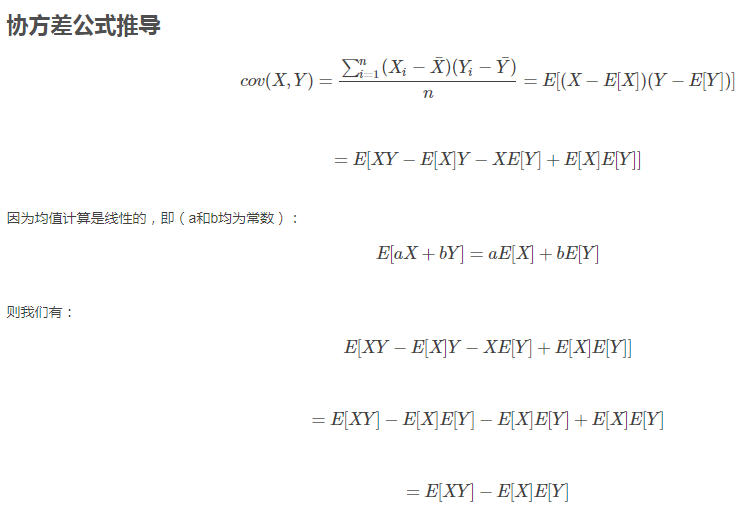转载于:https://www.cnblogs.com/wyl0514/p/11174103.html
展开全文• 以下资源均来自互联网马氏距离与其推导马氏距离就是用于度量两个坐标点之间的距离关系，表示数据的协方差距离。与尺度无关的(scale-invariant)，即独立于测量尺度。基本思想（intuition）如下图的过程（以两个维度...
以下资源均来自互联网

马氏距离与其推导

马氏距离就是用于度量两个坐标点之间的距离关系，表示数据的协方差距离。与尺度无关的(scale-invariant)，即独立于测量尺度。

基本思想（intuition）

如下图的过程（以两个维度作为例子），此例的数据重心为原点，P1,P2到原点的欧氏距离相同，但点P2在y轴上相对原点有较大的变异，而点P1在x轴上相对原点有较小的变异。所以P1点距原点的直观距离是比P2点的小的。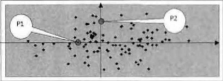马氏距离就是解决这个问题，它将直观距离和欧式距离统一。它先将数据不同维度上的方差统一（即各维度上的方差相同），此时的欧式距离就是直观距离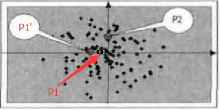如图：统一方差后的图，P1到原点的距离小于P2。P1’到原点的欧式距离和P2的相同。以上所说的直观距离就是马氏距离。但是，如果不同维度之间具有相关性，则压缩的效果就不好了。如下图只在横向和纵向上压缩，则达不到上图的压缩效果。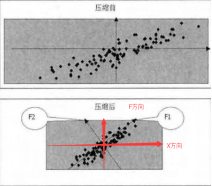所以在F1方向和F2方向上压缩数据才能达到较好的效果。所以需要将原始的在X坐标系中的坐标表示在F坐标系中。然后再分别沿着坐标轴压缩数据。

所以，计算样本数据的马氏距离分为两个步骤：
1. 坐标旋转
2. 数据压缩

坐标旋转的目标：使旋转后的各个维度之间线性无关，所以该旋转过程就是主成分分析的过程。 数据压缩的目标：所以将不同的维度上的数据压缩成为方差都是1的的数据集。

推导过程

有一个原始的多维样本数据Xn×mXn×m(m列，n行):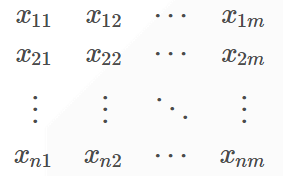其中每一行表示一个测试样本（共n个）；
Xi表示样本的第i个维度（共m个）Xi=(x1i　x2i　⋯　xni)T<!--//--><![CDATA[//><!--
Xi=(x_{1i}　x_{2i}　⋯　x_{ni})^T
//--><!]]>，以上多为样本数据记为X=(X1,X2⋯Xm)X=(X1,X2⋯Xm)。样本的总体均值为 μX=(μX1,μX2,⋯,μXm)<!--//--><![CDATA[//><!--
μ_X=(μ_{X1},μ_{X2},⋯,μ_{Xm})
//--><!]]>
其协方差为：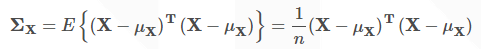协方差矩阵是在具有一组样本后，表示数据的各维度之间的关系的。其中n是样本的数量
假设将原始数据集X通过坐标旋转矩阵U旋转到新的坐标系统中得到一个新的数据集F。（其实X和F表示的是同一组样本数据集，只是由于其坐标值不同，为了易于区分用了两个字母表示）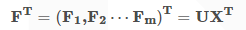新数据集F的均值记为μF=(μF1,μF2,⋯,μFm)<!--//--><![CDATA[//><!--
μ_F=(μ_{F1},μ_{F2},⋯,μ_{Fm})
//--><!]]>XμF=UμX<!--//--><![CDATA[//><!--
X_{μ_F}=U_{μ_X}
//--><!]]>

由于将数据集旋转后数据的各维度之间是不相关的，所以新数据集F的协方差矩阵ΣF<!--//--><![CDATA[//><!--
\Sigma_{F}
//--><!]]>应该为对角阵。

由于: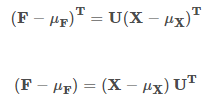所以：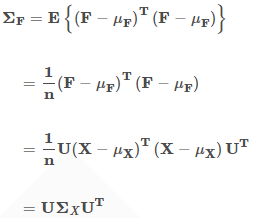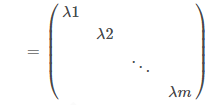每个λi−−√<!--//--><![CDATA[//><!--
\sqrt{\lambda_i}
//--><!]]>就是每个维度值方差。
由于ΣX<!--//--><![CDATA[//><!--
\Sigma_X
//--><!]]>是实对角阵，所以U是一个正交矩阵。
以上是准备知识，下面推导一个样本点x=(x1,x2⋯xm)到重心μX=(μX1,μX2⋯μXm)的马氏距离。等价于求点f=(f1,f2⋯fm)压缩后的坐标值到数据重心压缩后的坐标值μF=(μF1,μF2⋯μFm)的欧式距离。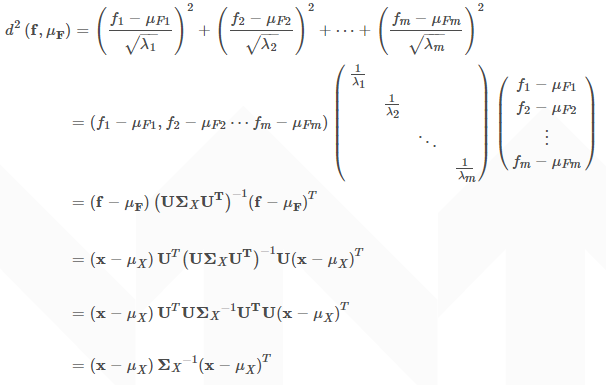这就是马氏距离的的计算公式了。
如果x是列向量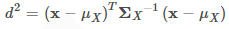如果并把上文的重心点μX=(μX1,μX2,⋯,μXm)<!--//--><![CDATA[//><!--
μ_X=(μ_{X1},μ_{X2},⋯,μ_{Xm})
//--><!]]>改为任意一个样本点y，则可以得到x和y两个样本点之间的马氏距离公式为：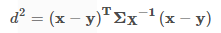以上来自这里

协方差公式

统计学的基本概念

学过概率统计的孩子都知道，统计里最基本的概念就是样本的均值，方差，或者再加个标准差。首先我们给你一个含有n个样本的集合X={X1,…,Xn}，依次给出这些概念的公式描述，这些高中学过数学的孩子都应该知道吧，一带而过。很显然，均值描述的是样本集合的中间点，它告诉我们的信息是很有限的，而标准差给我们描述的则是样本集合的各个样本点到均值的距离之平均。以这两个集合为例，[0，8，12，20]和[8，9，11，12]，两个集合的均值都是10，但显然两个集合差别是很大的，计算两者的标准差，前者是8.3，后者是1.8，显然后者较为集中，故其标准差小一些，标准差描述的就是这种“散布度”。之所以除以n-1而不是除以n，是因为这样能使我们以较小的样本集更好的逼近总体的标准差，即统计上所谓的“无偏估计”。而方差则仅仅是标准差的平方。

为什么需要协方差？

面几个统计量看似已经描述的差不多了，但我们应该注意到，标准差和方差一般是用来描述一维数据的，但现实生活我们常常遇到含有多维数据的数据集，最简单的大家上学时免不了要统计多个学科的考试成绩。面对这样的数据集，我们当然可以按照每一维独立的计算其方差，但是通常我们还想了解更多，比如，一个男孩子的猥琐程度跟他受女孩子欢迎程度是否存在一些联系啊，嘿嘿~协方差就是这样一种用来度量两个随机变量关系的统计量，我们可以仿照方差的定义：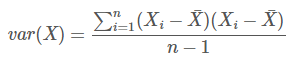来度量各个维度偏离其均值的程度，标准差可以这么来定义：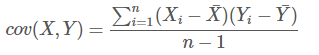协方差的结果有什么意义呢？如果结果为正值，则说明两者是正相关的(从协方差可以引出“相关系数”的定义)，也就是说一个人越猥琐就越受女孩子欢迎，嘿嘿，那必须的~结果为负值就说明负相关的，越猥琐女孩子越讨厌，可能吗？如果为0，也是就是统计上说的“相互独立”。

从协方差的定义上我们也可以看出一些显而易见的性质，如：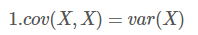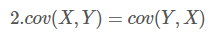协方差多了就是协方差矩阵

上一节提到的猥琐和受欢迎的问题是典型二维问题，而协方差也只能处理二维问题，那维数多了自然就需要计算多个协方差，比如n维的数据集就需要计算n!(n−2)!∗2<!--//--><![CDATA[//><!--
\frac{n!}{(n−2)!∗2}
//--><!]]>个协方差，那自然而然的我们会想到使用矩阵来组织这些数据。给出协方差矩阵的定义：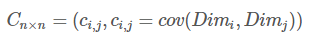这个定义还是很容易理解的，我们可以举一个简单的三维的例子，假设数据集有{x,y,z}{x,y,z}三个维度，则协方差矩阵为：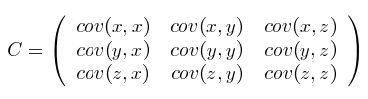可见，协方差矩阵是一个对称的矩阵，而且对角线是各个维度上的方差。

Matlab协方差实战

上面涉及的内容都比较容易，协方差矩阵似乎也很简单，但实战起来就很容易让人迷茫了。必须要明确一点，### 协方差矩阵计算的是不同维度之间的协方差，而不是不同样本之间的。这个我将结合下面的例子说明，以下的演示将使用Matlab，为了说明计算原理，不直接调用Matlab的cov函数。

首先，随机产生一个10*3维的整数矩阵作为样本集，10为样本的个数，3为样本的维数。

MySample = fix(rand(10,3)*50)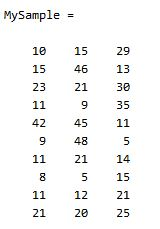根据公式，计算协方差需要计算均值，那是按行计算均值还是按列呢，我一开始就老是困扰这个问题。前面我们也特别强调了，协方差矩阵是计算不同维度间的协方差，要时刻牢记这一点。样本矩阵的每行是一个样本，每列为一个维度，所以我们要### 按列计算均值。为了描述方便，我们先将三个维度的数据分别赋值：

dim1 = MySample(:,1);
dim2 = MySample(:,2);
dim3 = MySample(:,3);

计算dim1与dim2，dim1与dim3，dim2与dim3的协方差：

sum( (dim1-mean(dim1)) .* (dim2-mean(dim2)) ) / ( size(MySample,1)-1 ) % 得到  74.5333
sum( (dim1-mean(dim1)) .* (dim3-mean(dim3)) ) / ( size(MySample,1)-1 ) % 得到  -10.0889
sum( (dim2-mean(dim2)) .* (dim3-mean(dim3)) ) / ( size(MySample,1)-1 ) % 得到  -106.4000

搞清楚了这个后面就容易多了，协方差矩阵的对角线就是各个维度上的方差，下面我们依次计算：

std(dim1)^2 % 得到   108.3222
std(dim2)^2 % 得到   260.6222
std(dim3)^2 % 得到   94.1778

这样，我们就得到了计算协方差矩阵所需要的所有数据，调用Matlab自带的cov函数进行验证：

cov(MySample)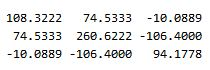把我们计算的数据对号入座，是不是一摸一样？
+++++++++++++++++++++++++++++++++++++++++++++++++++++++++++++++++

Update：今天突然发现，原来协方差矩阵还可以这样计算，先让样本矩阵中心化，即每一维度减去该维度的均值，使每一维度上的均值为0，然后直接用新的到的样本矩阵乘上它的转置，然后除以(N-1)即可。其实这种方法也是由前面的公式通道而来，只不过理解起来不是很直观，但在抽象的公式推导时还是很常用的！同样给出Matlab代码实现：
···
X = MySample - repmat(mean(MySample),10,1);    % 中心化样本矩阵，使各维度均值为0
C = (X’*X)./(size(X,1)-1);
···

总结
理解协方差矩阵的关键就在于牢记它计算的是不同维度之间的协方差，而不是不同样本之间，拿到一个样本矩阵，我们最先要明确的就是一行是一个样本还是一个维度，心中明确这个整个计算过程就会顺流而下，这么一来就不会迷茫了~

以上来自这里

无偏估计

https://www.zhihu.com/question/38185998
展开全文机器学习 无偏估计
• 首先附上协方差公式： 来设5个样本点：（3,9），（2,7），（4,12），（5,15），（6,17） 用R绘制出散点图，大概是这样： 要求这5个点的协方差，首先样本点为5个，n=5，X依次取3,2,4,5,6，Y依次取9,7,12,15,17。X的...r语言
• 公式： 假设数据集有三个维度x,y,z，则协方差矩阵为 常用：PCA降维中需要计算相关系数矩阵（即协方差矩阵） 获得协方差矩阵方法：cov是numpy库中计算协方差的函数，可直接调用获得协方差矩阵 data_zs_cov = np.cov...
• 已知n维随机变量$$\vec{X}=(X_{1},X_{2},...,X_{n})$$的协方差矩阵为\(C = \begin{bmatrix}c_{11} & c_{12} & ... & c_{1n} \\c_{21} & c_{22} & ... & c_{2n} \\. & .& &.\\. ...
• 协方差用于表示变量间的相互关系，变量间的相互关系一般有三种：正相关，负相关和不相关。 正相关：假设有两个变量x和y，若x越大y越...协方差公式如下（2个变量的情况下）：当维度多的时候可以用协方差矩阵来表示...
• 首先我们给你一个含有n个样本的集合，依次给出这些概念的公式描述，这些高中学过数学的孩子都应该知道吧，一带而过。 很显然，均值描述的是样本集合的中间点，它告诉我们的信息是很有限的，而标准差给我们...
• 1.协方差和协方差矩阵的概念公式1.1协方差公式1.2协方差矩阵公式有数据集={X,Y,Z}，是三维度的数据，即此此数据集中的样例有3个特征2.协方差的多种求解Python实现2.1代码2.2运行结果3.协方差矩阵的多种求解Python...
• 为什么需要协方差 学过概率论，我们知道均值，方差和标准差，但我们应该注意到，标准差和方差是用来描述一维数据的，现实生活中我们常常遇到含有多维数据的数据集，我们当然可以按照每一位独立的计算方差和标准差，...数据分析
• 要计算协方差，您需要类似下面这样的内容，它有一个嵌套循环，遍历每个列表，并使用协方差公式累积协方差。在# let's get the mean of X (add all the vals in X and divide by# the lengthx_mean = float(sum(X......